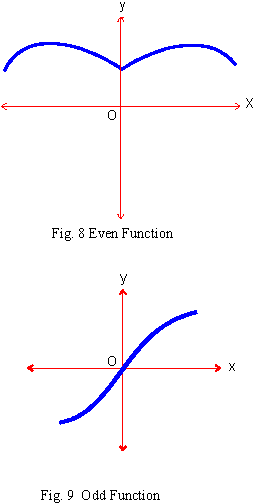Support the Monkey! Tell All your Friends and TeachersHome MonkeyNotes Printable Notes Digital Library Study Guides Study Smart Parents Tips College Planning Test Prep Fun Zone Help / FAQ How to Cite New Title Request

 5.3 Even & Odd (1) Even Function A function f (t) is said to be 'Even' when it satisfies the condition f (-t) = f (t) For example, cos (-t) = cos t, sec (-t) = sec t are even circular functions. It should be noted that the graph of an even function is symmetrical about the vertical. (i.e. y axis.) (2) Odd Function A function f (t) is said to be 'Odd' when it satisfies the condition f (-t) = -f (t) For example, sin (-t) = -sin t, csc (-t) = -csc tan (-t) = -tan t, cot (-t) = -cot (t) are 'odd' circular functions.It should be noted that the graph of an odd function is symmetrical about the origin of the rectangular coordinate system. (i) product of two even functions is an even function. (ii) product of two odd functions is an even function. (iii) product of an even and an odd function is an odd function. (iv) Any function f(t) can be expressed as the sum of two component functions of which one is even [f e(t)] and the other is odd [fo (t)] Thus f(t) = f e(t) + fo (t) (v) The sum of an odd and an even function is neither even nor odd. Index
 Search: All Products Books Popular Music Classical Music Video DVD Toys & Games Electronics Software Tools & Hardware Outdoor Living Kitchen & Housewares Camera & Photo Cell Phones Keywords: